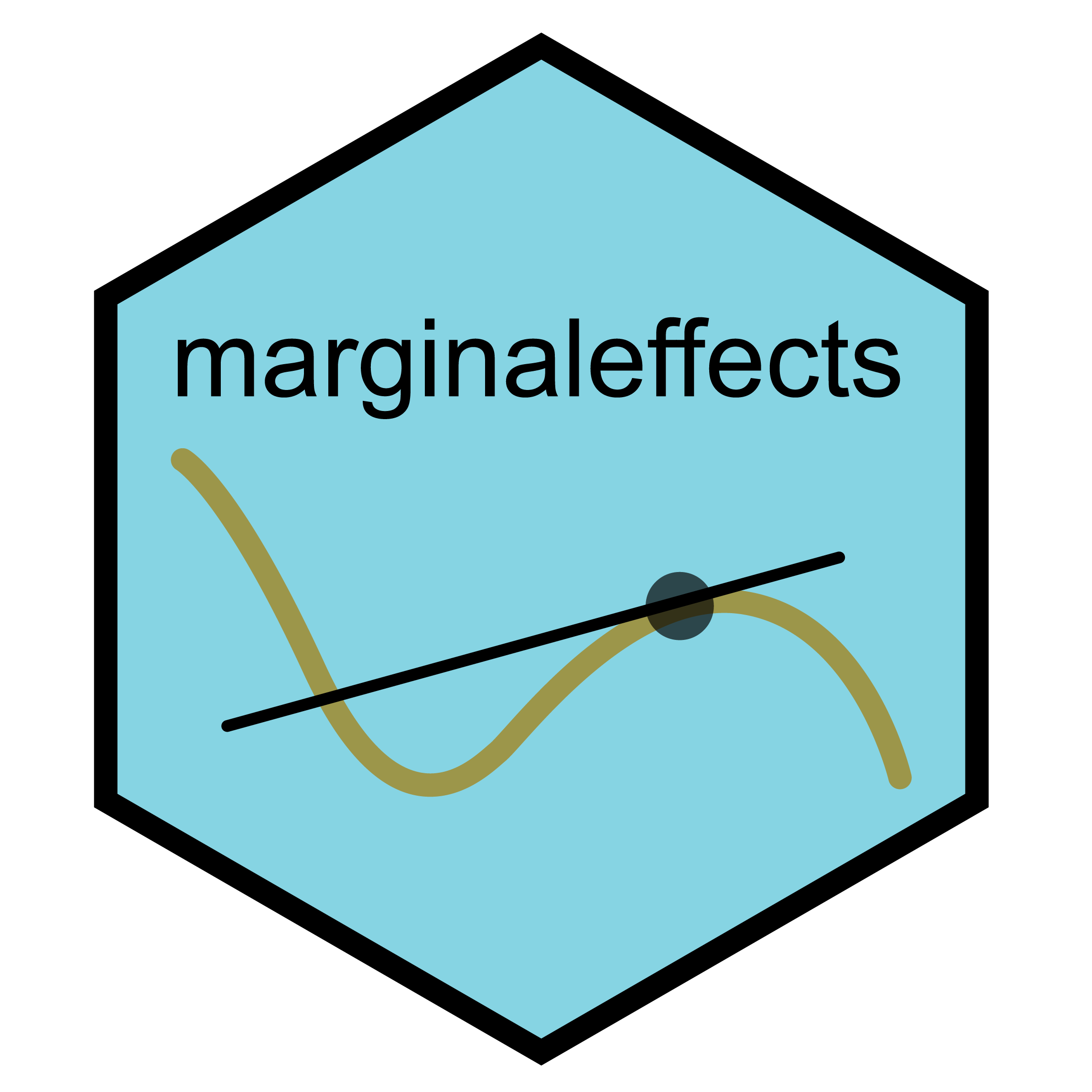# The `marginaleffects` package for `R``marginaleffects` allows users to compute and plot predictions, slopes, marginal means, and comparisons (contrasts, risk ratios, odds, etc.) for over 100 classes of statistical models. It can conduct linear and non-linear hypothesis tests, or equivalence tests, and calculate uncertainty estimates using the delta method, bootstrapping, or simulation-based inference.

The `marginaleffects` book (website) includes a “Get started” page, and over 25 chapters full of tutorials, case studies, and technical notes.

https://marginaleffects.com